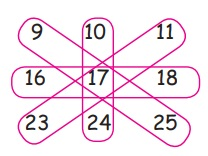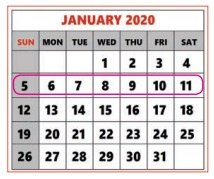Home | | Maths 4th Std | Use calendar (interlinking with patterns)

# Use calendar (interlinking with patterns)

Now we will learn how 60 is used in time

Now we will learn how 60 is used in time

60 seconds = 1 minute

60 minutes = 1 hour

1 hour = 60 × 60 = 3600 Seconds

24 hours = 1 day

Normally 12 hours is used. But in Railways, airlines, armed forces and TV channels 24 hours are used.

Now, learn the patterns in the Calendar.Consider 2 X 2 square in the nearby month

Add the numbers in the following way so that you will get the same total as 185 + 13 = 18

12 + 6 = 18

See the patterns in 3 X 3 square in the nearby month

Add the numbers in the following way so that you will get the same total as 519 + 17 + 25 = 51

23 + 17 +11 = 51,

16 + 17 + 18 = 51,

10 + 17 + 24 = 51

Note:

Calendar can teach us many things

1. In a row, number increases by 1

2. In a column, number increases by 7

Activity

Select any month and try to find 3 X 3 square and 2 X 2 square.

Let us see some more patterns in the following month.

Consider the line: 5 6 7 8 9 10 11Middle number is 8Sum of 5 and 11 is double the middle number (2x8) = 16

Sum of 6 and 10 is double the middle number (2x8) = 16

Sum of 7 and 9 is double the middle number (2x8) = 16

Try This

Select any month in a year. Find out the sum of any row and column. You wonder that the sum doubles the middle number.10+18+26 =54

24+18+12 =54

17+18+19 =54

11 + 18 +25 =546+14 =20

13+7 =20

Tags : Time | Term 3 Chapter 4 | 4th Maths , 4th Maths : Term 3 Unit 4 : Time
Study Material, Lecturing Notes, Assignment, Reference, Wiki description explanation, brief detail
4th Maths : Term 3 Unit 4 : Time : Use calendar (interlinking with patterns) | Time | Term 3 Chapter 4 | 4th Maths